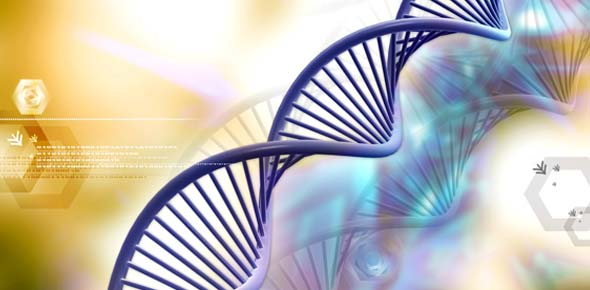# Ch.7-1 What Does DNA Look Like

10 Questions | Total Attempts: 289SettingsCh. 7 section 1 Quiz

Related Topics
• 1.
Deoxyribonucleic acid or ________________ is the material that contains the information that determines the traits a living thing inherits.
• A.

Nucleotide

• B.

DNA

• C.

Thymine

• D.

Cytosine

• 2.
____________________ is one of the four possible bases in a string of DNA and it pairs with adenine.
• A.

Nucleotide

• B.

DNA

• C.

Thymine

• D.

Cytosine

• 3.
__________________ is a subunit of DNA that consists of a sugar, a phosphate, and a nitrogenous base.
• A.

Nucleotide

• B.

DNA

• C.

Thymine

• D.

Cytosine

• 4.
_____________________ is the complementary base to guanine.
• A.

Nucleotid

• B.

DNA

• C.

Thymine

• D.

Cytosine

• 5.
What letters represent the four bases?
• A.

A, B, C, D

• B.

W, X, Y, Z

• C.

A, T, G, C

• D.

E, Y, A, O

• 6.
Using X-ray diffraction, what did Rosalind Franklin show the shape of DNA to be?
• A.

A circle

• B.

A spiral

• C.

A square

• D.

A line

• 7.
Watson and Crick built a DNA model like a
• A.

• B.

Piece of twine

• C.

Straight line

• D.

Pyramid

• 8.
• A.

Guanine and thymine.

• B.

• C.

Sugar and phosphate

• D.

Helixes and twists.

• 9.
The "rungs" of the DNA ladder are
• A.

A pair of bases

• B.

A pair of sugars

• C.

A pair of phosphates

• D.

A set of proteins

• 10.
To be copied, a DNA molecule splits
• A.

Across the top

• B.

Down the middle

• C.

Along the sides

• D.

Along the phosphates